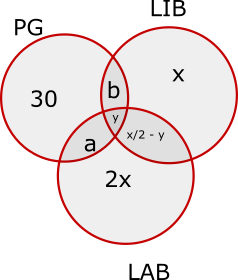### CAT 1991 Question Paper Question 26

Instructions

There were a hundred schools in a town. Of these, the number of schools having a play - ground was 30, and these schools had neither a library nor a laboratory. The number of schools having a laboratory alone was twice the number of those having a library only. The number of schools having a laboratory as well as a library was one-fourth the number of those having a laboratory alone. The number of schools having either a laboratory or a library or both was 35.

Question 26

# How many schools had none of the three viz., laboratory, library or play - ground?

SolutionThe diagram for this question has been shown:

Total number of schools having either or LAB or LIB or both = a+b+x/2 - y + y + 3x = 7x/2 + a + b = 35

Here a = b = y = 0

7x/2 = 35

x = 10

Total number of schools having at least one of PG, LIB or LAB = 30+2x+x+x/2 = 30+3x+x/2 = 30+30+5 = 65

Number of schools having neither of the three = 100-65 = 35

### View Video Solution

• All Quant CAT Formulas and shortcuts PDF
• 30+ CAT previous papers with solutions PDF

##### Kedar Lele

2 years, 6 months ago

Ok I understood ! It seems to come from the statement .... and these schools had neither a library nor a laboratory...I thought that it meant only G = 30 not that Other elements are zero !

Thanks

##### Kedar Lele

2 years, 6 months ago

Hi Sir For this Q How is the value of a& b and y 0 ?
Could you please explain that ? Thanks

OR